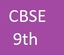Trending ▼   ResFinder# CBSE Class 9 Pre Board 2019 : Science (St Xavier's Sr. Sec. School, Delhi)

3 pages, 81 questions, 0 questions with responses, 0 total responses,00Cbse 9 +Fave Message ProfileTimelineUploads
 Home > cbse9 >   F Also featured on: School PageFormatting page ...

Annual Examination in SCIENCE Std. 9 19-2-2019 Time : 3 hrs. Max. Marks : 80 General Instructions: i) The question paper comprises of five sections A, B, C, D and E. You are to attempt all the sections. ii) All questions are compulsory. iii) Internal choice is given in sections B, C, D and E. iv) Question numbers 1 and 2 in Section-A are one mark questions. They are to be answered in one word or in one sentence. v) Question numbers 3 to 5 in Section- B are two marks questions. These are to be answered in about 30 words each. vi) Question numbers 6 to 15 in Section-C are three marks questions. These are to be answered in about 50 words each. vii) Question numbers 16 to 21 in Section-D are 5 marks questions. These are to be answered in about 70 words each. viii) Question numbers 22 to 27 in Section- E are based on practical skills. Each question is a two marks question. These are to be answered in brief. SECTION A 1. 2. Define displacement. Give an example where inspite of non-zero distance, displacement is zero. (1) Define : a) Atomicity b) Molecule (1) SECTION B 3. Draw a neat and well labeled diagram only to explain the observations of Rutherford s alpha ray scattering experiment. (2) 4. Differentiate between compost and vermicompost. (2) 5. Explain the following: a) Capture Fishery b) Apiculture (OR) What is animal husbandry? Differentiate between layers and broiler. (2) SECTION C 6. 7. 8. 9. a) b) What does slope of distance-time graph represent. Derive graphically, 2as=v2 - u2. Differentiate beetween g & G. A stone is released from top of tower 19.6 m. Calculate its final velocity just before touching the ground and time taken to reach the ground. (g = 9.8 m/s2) (OR) Differentiate between Thrust & Pressure. The volume of 50g of substance is 30 cm3. If density of water is 1 g/cm3. Calculate will the substance float or sink. (3) (3) a) b) c) What happens when acetone is poured on the palm? Explain. Suggest a method to liquefy gases. When 50g of sugar is dissolved in 100ml of water there is no increase in volume. Which characteristic of particles of matter is illustrated by this observation? (3) a) b) Differentiate between colloidal solution and suspension. (2 points) Which of the following materials fall in the category of a pure substance? i) Milk ii) Iron iii) Calcium Oxide To make a saturated solution 36g of common salt is dissolved in 100g of water at 293K. Find the concentration of the solution at this temperature. (OR) Differentiate between elements and compounds. Explain chemical change with the help of an example. Name the separation technique that you will apply for the following: i) Oil from water ii) Pigments from flowers (3) c) a) b) c)Formatting page ...Formatting page ...Tags : cbse class ix, cbse 9, cbse 9th standard, cbse mock papers, cbse sample papers, cbse books, portal for cbse india, cbse question bank, central board of secondary education, past cbse question papers with answers, prelims preliminary exams, pre board exam papers, cbse model test papers, solved board question papers of cbse last year, previous years solved question papers, free online cbse solved question paper, cbse syllabus, india cbse board sample questions papers, last 10 years cbse final exam papers, cbse question papers, cbse guess sample questions papers, cbse important questions, specimen / mock papers.